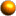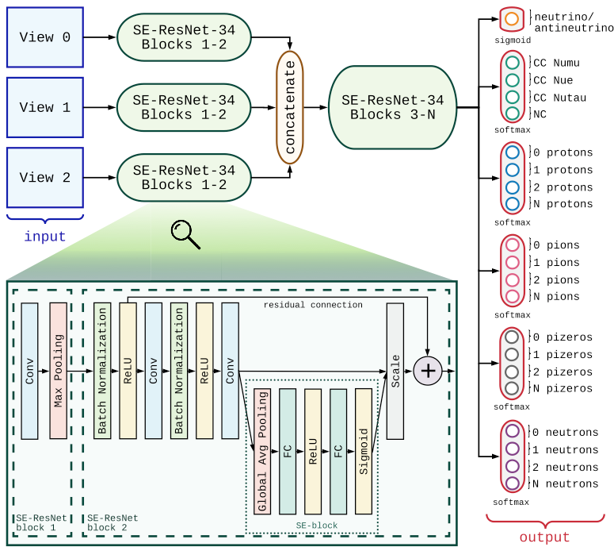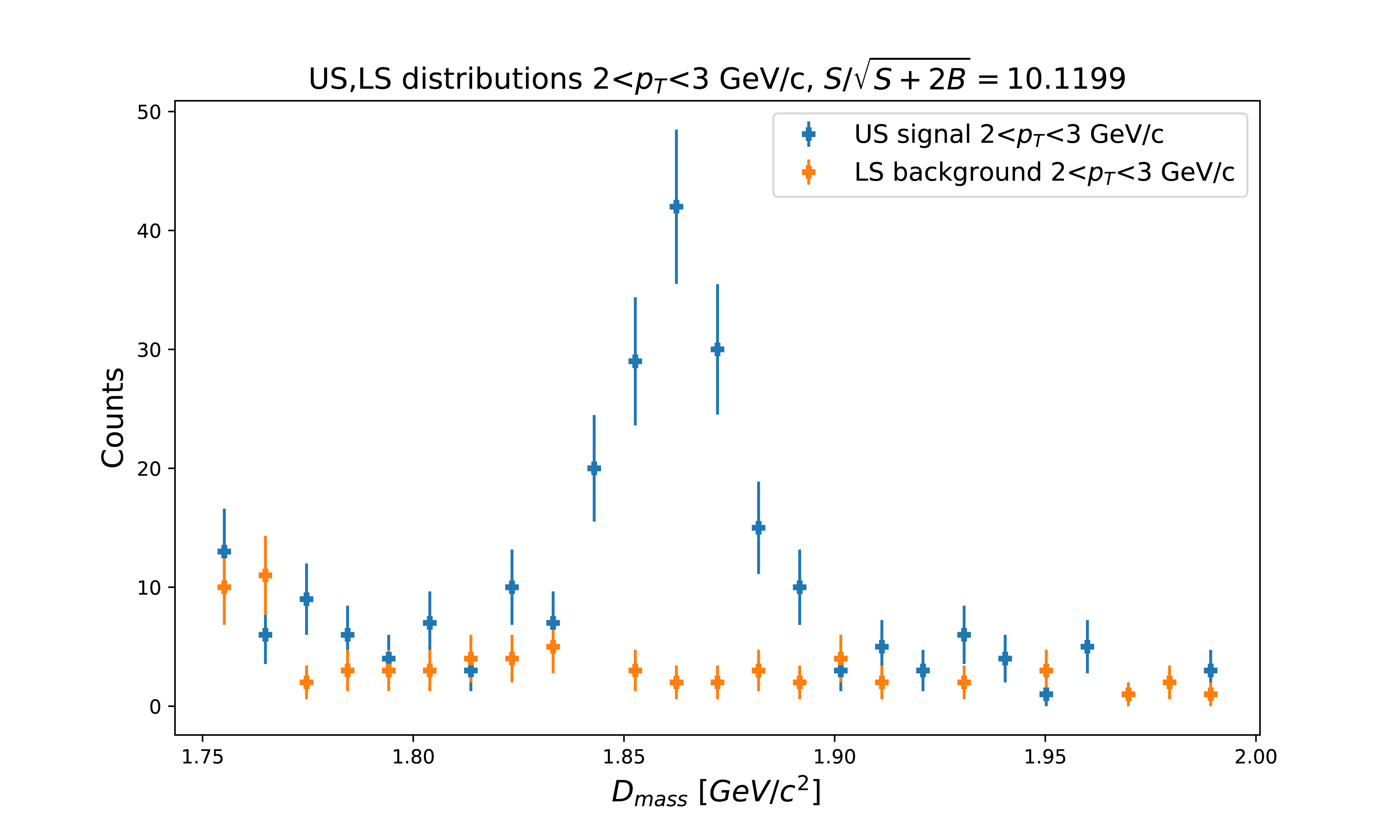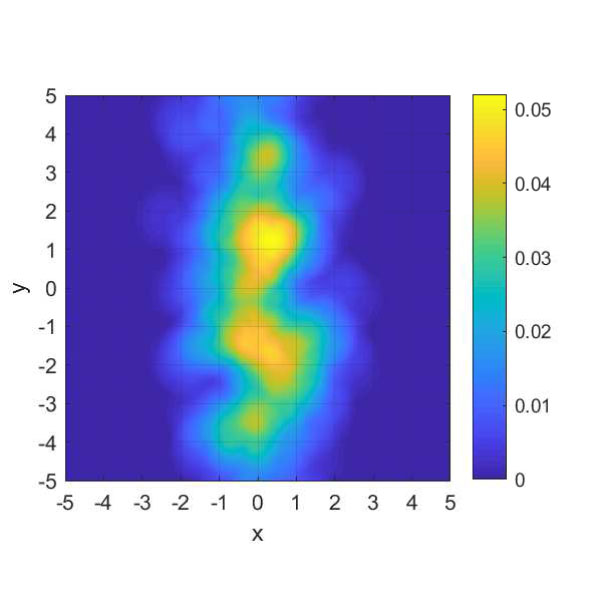# New methods of data selection and processing

 New statistical and non-statistical methods of data selection and processing are developed by a group of mathematicians from Department of Mathematics of the Faculty of Nuclear Sciences and Physical Engineering of the Czech Technical University (FJFI CVUT) and IT specialists from Institute of Computer Science of the Czech Academy of Sciences (UI AVCR).FJFI CVUT Group of mathematicians from FJFI CVUT develop special statistical and machine learning algorithms for specific classification problems which are key component of data analysis in particle physics. Convolutional and residual neural networks (CNN and ResNET) extract classification attributes from raw visual neutrino data for classification of neutrino interactions (NOvA/Fermilab). Optimization of decision trees (e.g. RF-Random Forest) with boosted improvements (AdaBoost, XGboost) Deep neural networks (DNN) separation of signal from heavy nuclei decays in d+Au collisions (STAR/BNL) Development of own supervised divergent decision tree (SDDT) using minimization of suitable non-metric distance (disparity) between non-parametric estimates of distributions in decision tree nodes. Data preprocessing based on statistical techniques of divergencies, non-parametric density estimates, variable homogeneity tests, etc.Optimization of residual classifier network architecture.Results of neural networks used to recognize D0 mesons by separation of correct charge combinations from combinatorial background.Kernel density estimates of a two-dimensional distribution without sphering.